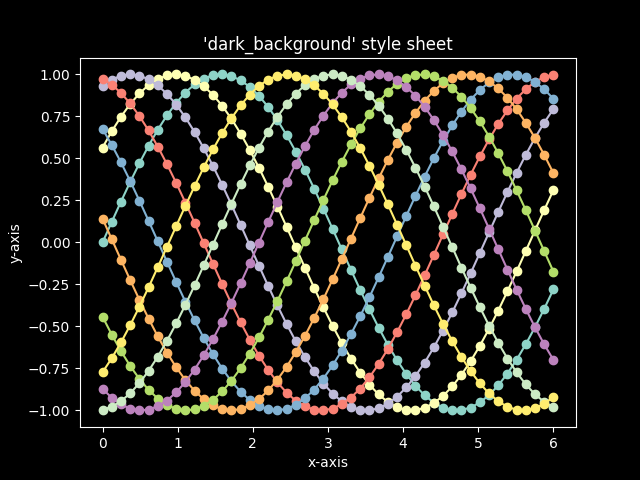# Dark background style sheet¶

This example demonstrates the "dark_background" style, which uses white for elements that are typically black (text, borders, etc). Note that not all plot elements default to colors defined by an rc parameter.import numpy as np
import matplotlib.pyplot as plt

plt.style.use('dark_background')

fig, ax = plt.subplots()

L = 6
x = np.linspace(0, L)
ncolors = len(plt.rcParams['axes.prop_cycle'])
shift = np.linspace(0, L, ncolors, endpoint=False)
for s in shift:
ax.plot(x, np.sin(x + s), 'o-')
ax.set_xlabel('x-axis')
ax.set_ylabel('y-axis')
ax.set_title("'dark_background' style sheet")

plt.show()


Keywords: matplotlib code example, codex, python plot, pyplot Gallery generated by Sphinx-Gallery